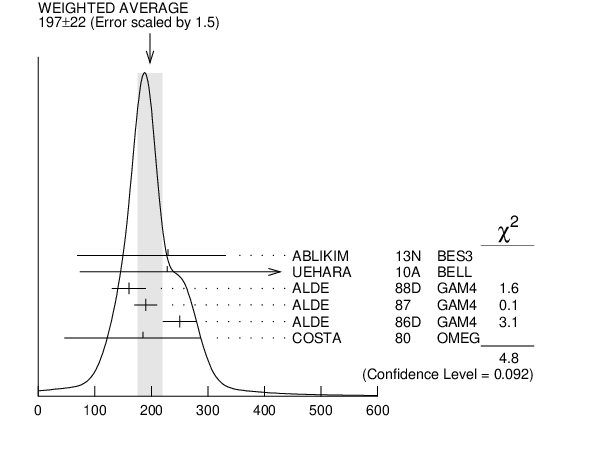# ${{\boldsymbol f}_{{2}}{(1810)}}$ WIDTH INSPIRE search

VALUE (MeV) EVTS DOCUMENT ID TECN  COMMENT
$\bf{ 197 \pm22}$ OUR AVERAGE  Error includes scale factor of 1.5.
$229$ ${}^{+52}_{-42}$ ${}^{+88}_{-155}$ 5.5k 1
 2013 N
BES3 ${{\mathit e}^{+}}$ ${{\mathit e}^{-}}$ $\rightarrow$ ${{\mathit J / \psi}}$ $\rightarrow$ ${{\mathit \gamma}}{{\mathit \eta}}{{\mathit \eta}}$
$228$ ${}^{+21}_{-20}$ ${}^{+234}_{-153}$ 2
 2010 A
BELL 10.6 ${{\mathit e}^{+}}$ ${{\mathit e}^{-}}$ $\rightarrow$ ${{\mathit e}^{+}}{{\mathit e}^{-}}{{\mathit \eta}}{{\mathit \eta}}$
$160$ $\pm30$ 40
 1988 D
GAM4 300 ${{\mathit \pi}^{-}}$ ${{\mathit p}}$ $\rightarrow$ ${{\mathit \pi}^{-}}{{\mathit p}}$4 ${{\mathit \pi}^{0}}$
$190$ $\pm20$ 1600
 1987
GAM4 100 ${{\mathit \pi}^{-}}$ ${{\mathit p}}$ $\rightarrow$ 4 ${{\mathit \pi}^{0}}{{\mathit n}}$
$250$ $\pm30$ 3
 1986 D
GAM4 100 ${{\mathit \pi}^{-}}$ ${{\mathit p}}$ $\rightarrow$ ${{\mathit \eta}}{{\mathit \eta}}{{\mathit n}}$
$185$ ${}^{+102}_{-139}$ 4
 1980
OMEG 10 ${{\mathit \pi}^{-}}$ ${{\mathit p}}$ $\rightarrow$ ${{\mathit K}^{+}}{{\mathit K}^{-}}{{\mathit n}}$
• • • We do not use the following data for averages, fits, limits, etc. • • •
$388$ ${}^{+15}_{-21}$ 5
 1986
RVUE Compilation
$280$ ${}^{+42}_{-35}$ 6
 1982
STRC 8 ${{\mathit \pi}^{+}}$ ${{\mathit p}}$ $\rightarrow$ ${{\mathit \Delta}^{++}}{{\mathit \pi}^{0}}{{\mathit \pi}^{0}}$
1  From partial wave analysis including all possible combinations of 0${}^{++}$, 2${}^{++}$, and 4${}^{++}$ resonances.
2  Breit-Wigner width. Could also be the $f_2(1910)$.
3  Seen in only one solution.
4  Error increased by spread of two solutions. Included in LONGACRE 1986 global analysis.
5  From a partial-wave analysis of data using a K-matrix formalism with 5 poles. Includes compilation of several other experiments.
6  From an amplitude analysis of the reaction ${{\mathit \pi}^{+}}$ ${{\mathit \pi}^{-}}$ $\rightarrow$ 2 ${{\mathit \pi}^{0}}$ . The resonance in the 2${{\mathit \pi}^{0}}$ final state is not confirmed by PROKOSHKIN 1997 .${{\mathit f}_{{2}}{(1810)}}$ width (MeV)
References:
 ABLIKIM 2013N
PR D87 092009 Partial Wave Analysis of ${{\mathit J / \psi}}$ $\rightarrow$ ${{\mathit \gamma}}{{\mathit \eta}}{{\mathit \eta}}$
 UEHARA 2010A
PR D82 114031 Measurement of ${{\mathit \eta}}{{\mathit \eta}}$ Production in Two-Photon Collisions
 ALDE 1988D
SJNP 47 810 Observation of the Tensor ${{\mathit X}{(1810)}}$ Meson in 300 GeV Central ${{\mathit \pi}^{-}}{{\mathit n}}$ Collisions
 ALDE 1987
PL B198 286 Neutral Mesons Decaying into 4${{\mathit \pi}^{0}}$
 ALDE 1986D
NP B269 485 Production of ${{\mathit G}{(1590)}}$ and other Mesons Decaying into ${{\mathit \eta}}$ Pairs by 100 ${\mathrm {GeV/}}\mathit c$ ${{\mathit \pi}^{-}}$ on Protons
 LONGACRE 1986
PL B177 223 A Measurement of ${{\mathit \pi}^{-}}$ ${{\mathit p}}$ $\rightarrow$ ${{\mathit K}_S^0}$ ${{\mathit K}_S^0}$ ${{\mathit n}}$ at 22 ${\mathrm {GeV/}}\mathit c$ and a Systematic Study of the $2+{}^{+}{}^{}$ Meson Spectrum
 CASON 1982
PRL 48 1316 Amplitude Analysis of the Reaction ${{\mathit \pi}^{+}}$ ${{\mathit \pi}^{-}}$ $\rightarrow$ ${{\mathit \pi}^{0}}{{\mathit \pi}^{0}}$ from 1.0 to 2.3 GeV
 COSTA 1980
NP B175 402 An Amplitude Analysis of the ${{\mathit K}^{+}}{{\mathit K}^{-}}$ System Produced in the Reaction ${{\mathit \pi}^{-}}$ ${{\mathit p}}$ $\rightarrow$ ${{\mathit K}^{+}}{{\mathit K}^{-}}{{\mathit n}}$ at 10 ${\mathrm {GeV/}}\mathit c$